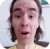FreeKB - Perl Count keys and values in a hash
Perl - Count keys and values in a hashEmpty hash

Let's say you have the following empty hash that contains no keys and no values.

``my %hash;``

The following can be used to print the number of keys and values in the hash.

``````my \$keys   = keys %hash;
my \$values = values %hash;
print "keys   = \$keys \n";
print "values = \$values \n";``````

Which should return the following.

``````keys = 0
values = 0``````

Hash with keys and values

Let's append some keys and values. In this example, the hash has three keys (employee_key, id_key, department_key) and three values (John Doe, 123456, Engineering)

``````my %hash;
\$hash{employee_key}   = "John Doe";
\$hash{id_key}         = "123456";
\$hash{department_key} = "Engineering";``````

Print the number of keys and values in the hash.

``````my \$keys   = keys %hash;
my \$values = values %hash;
print "keys   = \$keys \n";
print "values = \$values \n";``````

Which should return the following.

``````keys = 3
values = 3``````

Empty hash array

In this example, employees_key is an empty array, as indicated by the [ ] characters.

``my %hash = ( 'employees_key' => [] );``

Print the count of the array.

``````my \$count = @{\$hash{employees_key}};
print "count = \$count \n";``````

Which should return the following.

``count = 0``

Non-empty Hash array

Let's say you do this. Now the hash array contains two child keys (name_key and department_key).

``push @{\$hash{employees_key}}, { 'name_key' => 'John Doe', 'department_key' => 'engineering' };``

Print the count of the array.

``````my \$count = @{\$hash{employees_key}};
print "count = \$count \n";``````

Which should return the following.

``count = 1``

Child keys

The following can be used to print the number of child keys in the hash.

``````foreach my \$employees_key (@{\$hash{employees_key}}) {
my \$count = keys %\$employees_key;
print "\$count \n";
}``````

Which should return the following.

``2``

We will never share your name or email with anyone. Enter your email if you would like to be notified when we respond to your comment.

Please enter 68380 in the box below so that we can be sure you are a human.

Web design by yours truely - me, myself, and I   |   jeremy.canfield@freekb.net   |